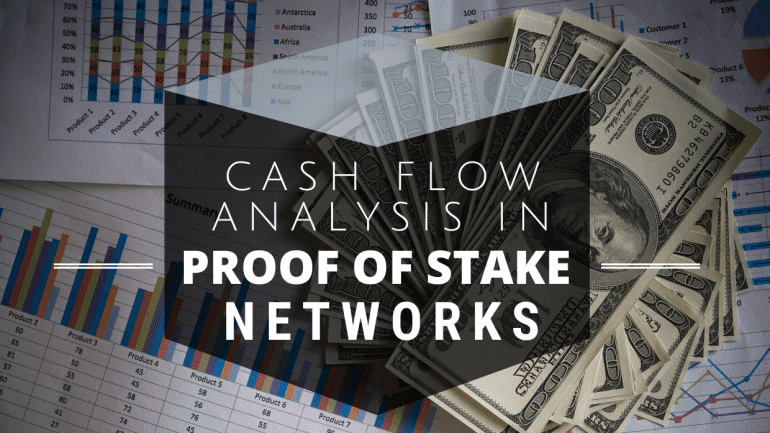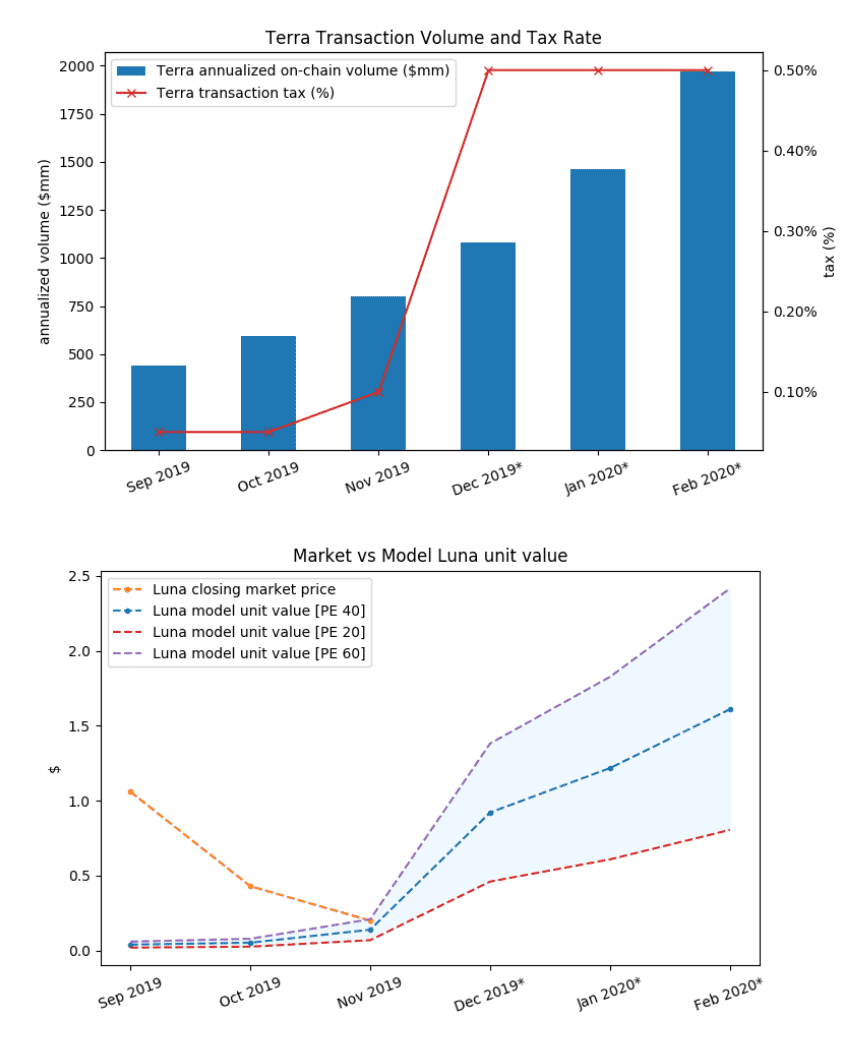BTC Bitcoin
 ETH Ethereum
 BCH Bitcoin Cash
 USDT Tether
 XLM Stellar
 XRP XRP
 LTC Litecoin
 CRO Crypto.com# Cash Flow Analysis in Proof of Stake Networks· in Crypto News
Carlos is an international relations' analyst specializing in cryptocurrencies and blockchain technology. Since 2017, Carlos has written extensively for UseTheBitcoin and other leading cryptocurrency sites; with over 2,000 articles published.
Register for UTB Token Exclusive Presale Access

It is a well-known fact that finding a fair price for digital assets remains difficult. Since we are dealing with a completely new class of assets, traditional valuation methods that are applied to stocks or foreign currency are often not applicable. For example, as cryptocurrencies act as a medium of exchange, many economists try to apply the Equation of Exchange (EoE) on crypto assets.

The EoE states that MV = PQ where M is the total supply of money, V is the velocity rate of exchange (i.e. how often the money supply is being turned over during a given time interval), P is the price level of an economy, and Q is the total quantity of goods and services produced. Hence, PQ is the nominal GDP, which is used when comparing the economic output of different countries, while Q is the inflation adjusted real GDP and MV is the total amount of spending.

The most common application of the EoE is in monetary theory, where it is used to explain the inflation rate. Under the assumption that V and Q are constant, an increase in the money supply by a certain percentage amount transfers to an equal inflation rate. Similarly, if V increases and M and Q stay constant, it means that people pay more money overall for the same amount of goods and services, whereas an increasing Q under constant VM means that there are more goods and services being sold, but people pay the same total amount (aka deflation). Well, duh!

Besides standing on the questionable theoretic ground of monetarist and keynesian economics, there are a number of problems when we try to apply the EoE to cryptocurrencies. The EoE formula MV = PQ is of course a tautology, since both MV and PQ are both methods to calculate the nominal GDP. However, while the GDP of a nation is a well-defined term, it is difficult to determine what transactions contribute to the “GDP” of a cryptocurrency. Most transactions in the cryptoeconomy simply exchange one digital asset for another.

For example, When you exchange BTC for another digital asset (such as ETH) or a physical good (such as the classic cup of coffee), the transaction should not contribute towards the Gross Domestic Product of Bitcoin, since neither ETH nor coffee are domestic goods of Bitcoin. However, these transactions undoubtedly contribute towards Bitcoin’s value, since they make use of Bitcoin’s function as a medium of exchange.

The EoE only regards money as a means of exchange and not as a store of value, which is one of Bitcoin’s other purposes. Whenever there are HODLers who speculate on the rising value of a currency, the EoE breaks down. On top of that, crypto markets have a comparably low liquidity and sudden changes in supply and demand can make a large and often unforeseen impact on asset prices.

## Market Capitalization and Cash Flow

Rather than trying to calculate some sort of GDP, treating cryptocurrency units like stocks seem like a more sensible approach. For this reason, market capitalization (token price multiplied by the number of tokens in circulation) is the prime method for comparing the economic strength of different assets. The traditional approach to valuing stocks is a cash flow analysis. In essence, the surplus that a publicly traded company earns is distributed to shareholders either by dividends or by retaining the surplus for future investments that increase the company’s market cap.

Similar to the GDP approach, in crypto assets it is often difficult to identify cash flows that contribute to the market cap. One exception for this is the payment network Terra, which is a stablecoin payment network that levies a transaction fee on payments that is redistributed to stakers who secure the network by staking Luna tokens. Since Terra can make predictions about the future increase of transactions value and the total transaction fees that are paid out to stakers, it is possible to calculate a fair token price using the same methods as for stocks.

Namely, Terra uses the price-to-earnings ratio, also called earnings multiple for valuation, which essentially denotes after how many years of consecutive earnings the total earnings match the token price. The earnings multiple gives investors an indication on how much they have to pay in order to obtain a share, or token in this case, that grants them a fixed annual return.Terra assumes an average compound growth rate in transaction volume of 35% over the next few months (top). The resulting token valuation is modeled using an earnings multiple of 20, 40, and 60 (bottom).

While the Terra network is an exceptional case as the supply of Luna is fixed, most Proof of Stake networks reward stakers by minting new tokens at a certain annual rate, which of course dilute the value of tokens, just like increasing the money supply dilutes the value of currency units. Rather than using a straight forward cash flow analysis, we therefore need to introduce the concept of network equity. Your equity in a crypto network is the number of asset units that you hold, divided by the number of circulating units. Resolving the formula to calculate the market cap (C = p * M) where p denotes the price of one unit and M being again the total number of units (money supply), this means that p = C / M. In other words, the equity of one token at price p equals the market cap divided by the number of circulating units.

Now, when new tokens are minted or mined over a span of time, the network equity of singular tokens naturally drops. Assuming that the market cap stays the same, you can calculate the price of one unit by adjusting M in the equation to accomodate for the new supply. However, when you stake tokens, this will earn you more tokens as a reward.

You might now assume that your network equity stays the same when staking tokens, as you receive an annual return of some percent on your stake, but the supply of units increases by the same percentage. This would be true in a network where all tokens are staked, but in reality, some users will not stake their tokens but hold them aside for transactions, paying for network services, or for speculation. Actually, your network equity therefore grows, as only the unstaked tokens get diluted. One might say that, in contrast to Luna’s cash flow, there is a flow of network equity from the holders of unstaked tokens toward the holders of staked tokens.

Let Mu be the number of unstaked tokens and Ms be the number of staked tokens, where M = Mu + Ms in a PoS network that gives staked tokens an annual return of r. For ease of the equation, the annual return factors in the original investment, so if the network offers annual staking rewards of 6%, then r = 1.06. Furthermore, we will disregard any compound effects in our analysis. If we assume that the ratio between staked and unstaked units remains the same over the course of one year, this means that after the year we will have a total supply of M’ = Mu + rMs.

This in turn lets us calculate how the network equity of a single token looks like after one year. Remember that the network equity of a single unit is the inverse number of total units (E = 1 / Mu + Ms). This means that after one year of distributing staking rewards, the equity of a single unit E’ = 1 / (Mu + rMs). While this delutes the value of all tokens, stakers receive a reward factor of r on their stake. In terms of equity, this transfers into a total equity of Es’ = r / (Mu + rMs).

Assuming no change in market cap, the token price drops from level p = C / (Mu + Ms) to p’ = C / (Mu + rMs). The staker has therefore realized a total return of rp’ – p per token owned. This return, multiplied by the staker’s desired earnings multiple is the token price she would accept as a fair price.

## Conclusion

While using monetary theory to value digital assets has severe shortcomings, assets that grant their holders a discernable positive cash flow can be valued by multiplying the cash flow with the desired earnings multiple. While this is a straight forward process for assets with a direct cash flow such as Luna, most Proof of Stake networks distribute rewards through token dilution.

Rather than a cash flow, this creates a network equity flow from unstaked tokens towards staked ones. This lets stakers estimate their annual return under the assumption of an unchanging market cap. The volatility of token prices however tells us that this assumption often does not hold true. For comparing different investment opportunities in PoS networks, investors can introduce a discount rate to this cash flow analysis in order to accomodate for risks and potential additional earnings through changes in the market cap.